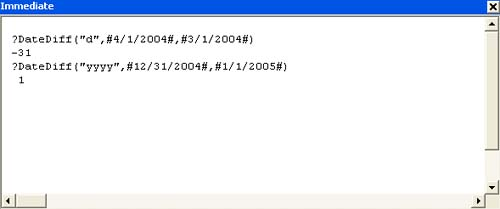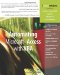# Working with Date Functions

 < Day Day Up >

Working with dates can be difficult if you don't know what you're doing. VBA won't help you use dates correctly if you don't know the basics. However, if you use dates in Access expressions (queries, calculated controls, and so on) without trouble, you should experience the same success using VBA. This section reviews a number of functions that work with dates.

CAUTION

All the date functions in this section are limited to the Date data type range restrictions. The first date value recognized is January 1, 100, and the last is December 31, 9999. Any date out of this range returns an error.

#### Returning the Date

VBA's Date function takes the simplest form of all

` `

` Date `

and returns a Variant Date subtype that equals the system's current date. (The Date\$ function returns a String data type.)

TIP

The Date function returns only the system's current date. If you need both the date and time, use the Now function.

To reset the system's current date, use the Date statement, in the following form

` `

` Date = newdate `

where newdate is a String, Date, or Variant Date subtype. For example, the statement

` `

` Date = "March 1, 2004" `

sets your system's date to March 1, 2004. However, it's better not use this method unless you have a specific reason for doing so. Changing your system's date can have far-reaching and unintended consequences.

#### Adding to and Subtracting from Dates

Use the DateAdd function to add or subtract a given number of time periods to or from a specific date. For instance, you might calculate the date that's 10 days or 10 months into the future (or in the past). Or, you might return the date and time 36 hours into the future.

This function takes the form

` `

` DateAdd(interval, number, date) `

where interval is a String value or expression that denotes the type of time period (day, week, or month) that you want to add or subtract. Table 5.1 lists the predefined interval settings. The number argument is a numeric value that specifies the number of times interval should be added or subtracted and date is a Date Variant that represents the date to which you're adding or subtracting interval.

##### Table 5.1. Interval String Settings

String Setting

Description

yyyy

Year

q

Quarter

m

Month

y

Day of year

d

Day

w

Weekday

ww

Week

h

Hour

n

Minute

s

Second

A negative interval value returns a date from the past; a positive value returns a date in the future. If number contains a fractional component, DateAdd rounds that value to the nearest whole number before actually calculating the function's results.

#### Determining the Difference Between Two Dates

To determine the number of time intervals between two dates, use VBA's DateDiff function. This function returns a Variant Long subtype and takes the form

` `

` DateDiff(interval, date1, date2[, firstdayofweek[, firstweekofyear]]) `

where interval is a String value that represents the type of time period to calculate between date1 and date2 (refer to Table 5.1). The two optional arguments, firstdayofweek and firstweekofyear, are numeric constants that define the first day of the week and the first day of the year, respectively. See Tables 5.2 and 5.3 for a list of constants. If omitted, these arguments default to Sunday and January 1, respectively.

##### Table 5.2. First Day of Week Constants

Constant

Description

Integer Value

vbSunday

Sunday (the default)

1

vbMonday

Monday

2

vbTuesday

Tuesday

3

vbWednesday

Wednesday

4

vbThursday

Thursday

5

vbFriday

Friday

6

vbSaturday

Saturday

7

##### Table 5.3. First Week of Year Constants

Constant

Description

Integer Value

vbFirstJan1

Use the week in which January 1 occurs (the default)

1

vbFirstFourDays

Use the first week that has at least four days in the new year

2

vbFirstFullWeek

Use the first full week of the new year

3

This function might not always yield the expected dates:

• When date2 falls before date1, the returned value is negative.

• Even though there's only one day between December 31 of one year and January 1 of the next, DateDiff knows there's an entire year between the two dates. This also happens when calculating the number of months and quarters.

Figure 5.7 shows these guidelines in action in the Immediate window.

##### Figure 5.7. Be mindful of DateDiff's specific calculation behaviors.NOTE

The dates in Figure 5.7 are enclosed by two pound sign characters (#). This character is the appropriate delimiter for date values, just as the quotation mark is the delimiter for string values. When using dates, you must delimit the value or Access will return an error or return erroneous data.

#### Extracting Date Components

It's easy to determine a specific date component using the DatePart function. For instance, the following functions return the values 4, 1, and 2004, respectively:

` `

` DatePart("m",#4/1/2004#) DatePart("d",#4/1/2004#) DatePart("yyyy",#4/1/2004#) `

This function returns a variant of the Integer subtype and takes the form

` `

` DatePart(interval, date[, firstdayofweek[, firstweekofyear]]) `

where interval is a String value that specifies the component you want to extract and date is a variant of the Date subtype and equals the date from which you're extracting interval. (Refer to Table 5.1 for the interval values.) The two optional arguments, firstdayofweek and firstweekofyear, are numeric constants that define the first day of the week and the first day of the year, respectively (see Tables 5.2 and 5.3 for a list of constants). If omitted, these arguments default to Sunday and January 1, respectively.

#### Creating a Date from Individual Components

In the last section, you learned how DatePart extracts a date component from a date. Similarly, you can use DateSerial to combine date components to create a date. This function returns a variant of the Date subtype using the form

` `

` DateSerial(year, month, day) `

where year, month, and day represent integer values that represent the corresponding date part. There are a few rules about the values you can use for each argument:

• year is required and must equal a numeric value from 100 to 9999.

• month is required and must be a numeric value from 1 to 12.

• day is required and must be a numeric value from 1 to 31.

TIP

Like most date functions, DateSerial assumes a year value between 0 and 29 is a 21st century date. Likewise, a year value between 30 and 99 is a 20th century date. To be safe, it's best to use four-digit values to express year values. It's always better to explicitly force date values rather than letting VBA guess.

If any of the argument values fall out of their respective ranges, DateSerial will just keep going into the next time period. For instance, the function

` `

` DateSerial(2004, 15, 3) `

returns March 3, 2005 because the 15th month of 2004 is March 2005. That is, DateSerial uses up the 12 months in 2004 and then goes three more months into 2005. Similarly,

` `

` DateSerial(2005, 4, 45) `

returns May 15, 2005. VBA uses up the 30 days in April and then goes 15 days more into May.

#### Creating a Date from a String Expression

VBA lets you create a date from a string expression. The DateValue function returns a Variant (Date subtype) and uses the form

` `

` DateValue(stringexpression) `

where stringexpression is required and uses the system's short date setting. In addition, the date separators must conform to those used by the system's settings. Time information is ignored; if only time values are used, the function returns an error. All three of the following functions return the same date, March 1, 2004:

` `

` DateValue("3/1/2004") DateValue("March 1, 2004") DateValue("1 Mar 04") `

TIP

The TimeSerial and TimeValue functions work similarly to the DateSerial and DateValue functions with time components.

#### Returning a Specific Date or Time Component

There are several functions that return specific date and time components. They're easy to use because all you need to specify is the date. Table 5.4 lists these functions.

##### Table 5.4. Date Component Functions

Function

Result

Day(date)

Returns an Integer value between 1 and 31 that represents a day of the month.

Hour(time)

Returns an Integer value between 0 and 23 that represents an hour of the day.

Minute(time)

Returns an Integer value between 0 and 59 that represents a minute.

Second(time)

Returns an Integer value between 0 and 59 that represents a second.

Month(date)

Returns an Integer value between 1 and 12 that represents a month.

Year(date)

Returns an Integer value that represents a year.

##### A Date Function Example

Have you ever needed to know the number of days you have to complete a project? Using the following function procedure, you can enter the project's beginning and ending dates and get an immediate response:

` `

` Public Function GetDays() As Integer   Dim dteStart As Date   Dim dteEnd As Date   Dim varDays As Variant   dteStart = InputBox("Enter beginning date")   dteEnd = InputBox("Enter ending date")   varDays = DateDiff("d", dteStart, dteEnd)   GetDays = varDays End Function `

1. First, enter the preceding function procedure in Chapter 5's example module.

2. In the Immediate window, enter the following statement and press Enter:

` `

` ?GetDays `

3. When VBA displays the first input box, enter 3/1/2004 and click OK.

4. In the second input box, enter 4/1/2004 and click OK.

##### Figure 5.8. Determining the number of days between two dates.< Day Day Up >Automating Microsoft Access with VBA
ISBN: 0789732440
EAN: 2147483647
Year: 2003
Pages: 186